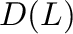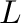# Blaise Bleriot Koguep Njionou – Derivation on Hyperlattices revisited

Department of Mathematics and Computer Science, Faculty of Science, University of Dschang
The main goal of this paper is to redefine the concept of derivation on hyperlattice. We introduce the notion of joinitive, strong-joinitive derivation and differential ideal in hyperlattice and study some of their properties and relation between them. Moreover, we prove that the setof all principal derivations on a bounded distributive hyperlatticeis a bounded distributive hyperlattice andis isomorphic to L. We also prove that given a strong-joinitive derivation d on a hyperlattice with a bottom element, the setof d-invariant ideals ofis lattice.